10.7 Parametric equations: graphs  (Page 3/4)

 Page 3 / 4

Access the following online resource for additional instruction and practice with graphs of parametric equations.

Key concepts

• When there is a third variable, a third parameter on which $\text{\hspace{0.17em}}x\text{\hspace{0.17em}}$ and $\text{\hspace{0.17em}}y\text{\hspace{0.17em}}$ depend, parametric equations can be used.
• To graph parametric equations by plotting points, make a table with three columns labeled $\text{\hspace{0.17em}}t,x\left(t\right),\text{\hspace{0.17em}}$ and $\text{\hspace{0.17em}}y\left(t\right).\text{\hspace{0.17em}}$ Choose values for $\text{\hspace{0.17em}}t\text{\hspace{0.17em}}$ in increasing order. Plot the last two columns for $\text{\hspace{0.17em}}x\text{\hspace{0.17em}}$ and $\text{\hspace{0.17em}}y.\text{\hspace{0.17em}}$ See [link] and [link] .
• When graphing a parametric curve by plotting points, note the associated t -values and show arrows on the graph indicating the orientation of the curve. See [link] and [link] .
• Parametric equations allow the direction or the orientation of the curve to be shown on the graph. Equations that are not functions can be graphed and used in many applications involving motion. See [link] .
• Projectile motion depends on two parametric equations: $\text{\hspace{0.17em}}x=\left({v}_{0}\mathrm{cos}\text{\hspace{0.17em}}\theta \right)t\text{\hspace{0.17em}}$ and $\text{\hspace{0.17em}}y=-16{t}^{2}+\left({v}_{0}\mathrm{sin}\text{\hspace{0.17em}}\theta \right)t+h.\text{\hspace{0.17em}}$ Initial velocity is symbolized as $\text{\hspace{0.17em}}{v}_{0}.\text{\hspace{0.17em}}\theta$ represents the initial angle of the object when thrown, and $\text{\hspace{0.17em}}h\text{\hspace{0.17em}}$ represents the height at which the object is propelled.

Verbal

What are two methods used to graph parametric equations?

plotting points with the orientation arrow and a graphing calculator

What is one difference in point-plotting parametric equations compared to Cartesian equations?

Why are some graphs drawn with arrows?

The arrows show the orientation, the direction of motion according to increasing values of $\text{\hspace{0.17em}}t.$

Name a few common types of graphs of parametric equations.

Why are parametric graphs important in understanding projectile motion?

The parametric equations show the different vertical and horizontal motions over time.

Graphical

For the following exercises, graph each set of parametric equations by making a table of values. Include the orientation on the graph.

$\left\{\begin{array}{l}x\left(t\right)=t\hfill \\ y\left(t\right)={t}^{2}-1\hfill \end{array}$

 $t$ $x$ $y$ $-3$ $-2$ $-1$ $0$ $1$ $2$ $3$

$\left\{\begin{array}{l}x\left(t\right)=t-1\hfill \\ y\left(t\right)={t}^{2}\hfill \end{array}$

 $t$ $-3$ $-2$ $-1$ $0$ $1$ $2$ $x$ $y$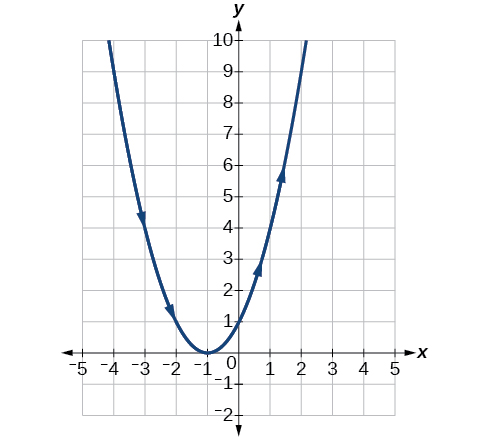$\left\{\begin{array}{l}x\left(t\right)=2+t\hfill \\ y\left(t\right)=3-2t\hfill \end{array}$

 $t$ $-2$ $-1$ $0$ $1$ $2$ $3$ $x$ $y$

$\left\{\begin{array}{l}x\left(t\right)=-2-2t\hfill \\ y\left(t\right)=3+t\hfill \end{array}$

 $t$ $-3$ $-2$ $-1$ $0$ $1$ $x$ $y$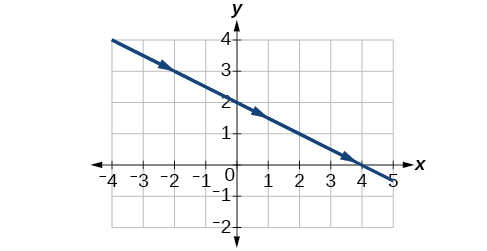$\left\{\begin{array}{l}x\left(t\right)={t}^{3}\hfill \\ y\left(t\right)=t+2\hfill \end{array}$

 $t$ $-2$ $-1$ $0$ $1$ $2$ $x$ $y$

$\left\{\begin{array}{l}x\left(t\right)={t}^{2}\hfill \\ y\left(t\right)=t+3\hfill \end{array}$

 $t$ $-2$ $-1$ $0$ $1$ $2$ $x$ $y$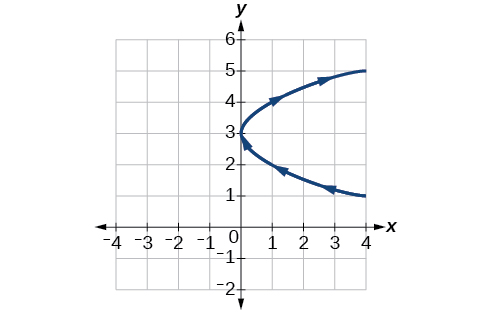For the following exercises, sketch the curve and include the orientation.

$\left\{\begin{array}{l}x\left(t\right)=t\\ y\left(t\right)=\sqrt{t}\end{array}$

$\left\{\begin{array}{l}x\left(t\right)=-\text{\hspace{0.17em}}\sqrt{t}\\ y\left(t\right)=t\end{array}$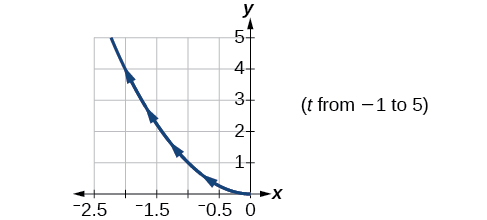$\left\{\begin{array}{l}x\left(t\right)=5-|t|\\ y\left(t\right)=t+2\end{array}$

$\left\{\begin{array}{l}x\left(t\right)=-t+2\\ y\left(t\right)=5-|t|\end{array}$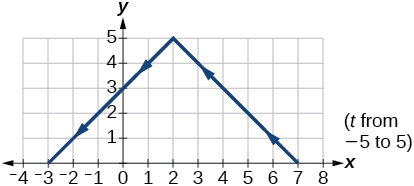$\left\{\begin{array}{l}x\left(t\right)=4\text{sin}\text{\hspace{0.17em}}t\hfill \\ y\left(t\right)=2\mathrm{cos}\text{\hspace{0.17em}}t\hfill \end{array}$

$\left\{\begin{array}{l}x\left(t\right)=2\text{sin}\text{\hspace{0.17em}}t\hfill \\ y\left(t\right)=4\text{cos}\text{\hspace{0.17em}}t\hfill \end{array}$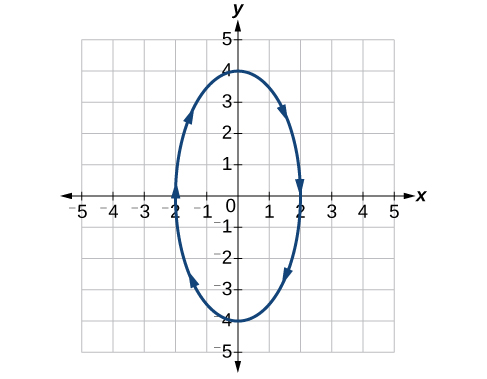$\left\{\begin{array}{l}x\left(t\right)=3{\mathrm{cos}}^{2}t\\ y\left(t\right)=-3\mathrm{sin}\text{\hspace{0.17em}}t\end{array}$

$\left\{\begin{array}{l}x\left(t\right)=3{\mathrm{cos}}^{2}t\\ y\left(t\right)=-3{\mathrm{sin}}^{2}t\end{array}$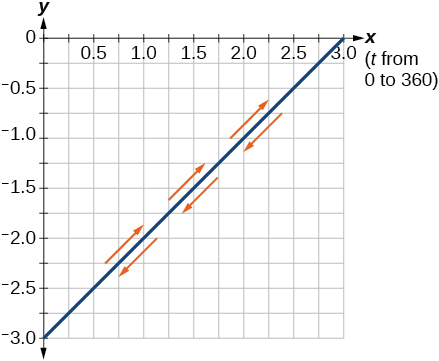$\left\{\begin{array}{l}x\left(t\right)=\mathrm{sec}\text{\hspace{0.17em}}t\\ y\left(t\right)=\mathrm{tan}\text{\hspace{0.17em}}t\end{array}$

$\left\{\begin{array}{l}x\left(t\right)=\mathrm{sec}\text{\hspace{0.17em}}t\\ y\left(t\right)={\mathrm{tan}}^{2}t\end{array}$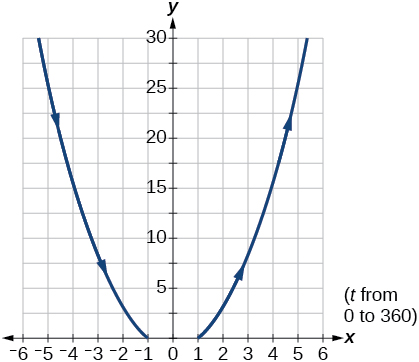$\left\{\begin{array}{l}x\left(t\right)=\frac{1}{{e}^{2t}}\\ y\left(t\right)={e}^{-\text{\hspace{0.17em}}t}\end{array}$

For the following exercises, graph the equation and include the orientation. Then, write the Cartesian equation.

$\left\{\begin{array}{l}x\left(t\right)=t-1\hfill \\ y\left(t\right)=-{t}^{2}\hfill \end{array}$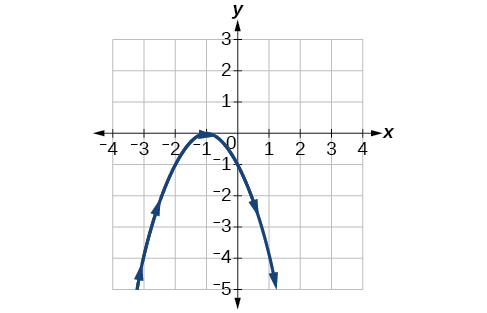$\left\{\begin{array}{l}x\left(t\right)={t}^{3}\hfill \\ y\left(t\right)=t+3\hfill \end{array}$

$\left\{\begin{array}{l}x\left(t\right)=2\mathrm{cos}\text{\hspace{0.17em}}t\\ y\left(t\right)=-\mathrm{sin}\text{\hspace{0.17em}}t\end{array}$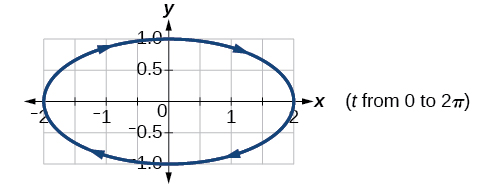$\left\{\begin{array}{l}x\left(t\right)=7\mathrm{cos}\text{\hspace{0.17em}}t\\ y\left(t\right)=7\mathrm{sin}\text{\hspace{0.17em}}t\end{array}$

$\left\{\begin{array}{l}x\left(t\right)={e}^{2t}\\ y\left(t\right)=-{e}^{\text{\hspace{0.17em}}t}\end{array}$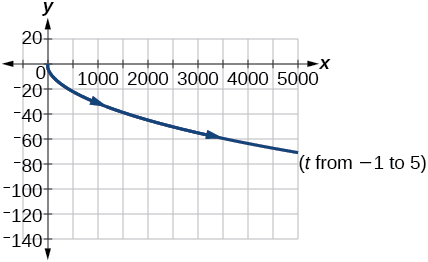For the following exercises, graph the equation and include the orientation.

$x={t}^{2},\text{\hspace{0.17em}}y\text{\hspace{0.17em}}=\text{\hspace{0.17em}}3t,\text{\hspace{0.17em}}0\le t\le 5$

$x=2t,\text{\hspace{0.17em}}y\text{\hspace{0.17em}}=\text{\hspace{0.17em}}\text{\hspace{0.17em}}{t}^{2},\text{\hspace{0.17em}}-5\le t\le 5$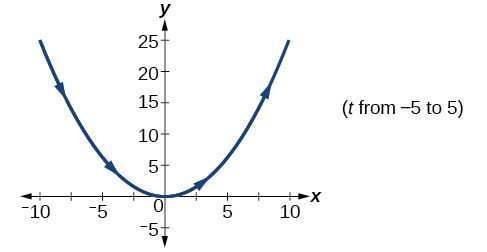$x=t,\text{\hspace{0.17em}}y=\sqrt{25-{t}^{2}},\text{\hspace{0.17em}}0

$x\left(t\right)=-t,y\left(t\right)=\sqrt{t},\text{\hspace{0.17em}}t\ge 0$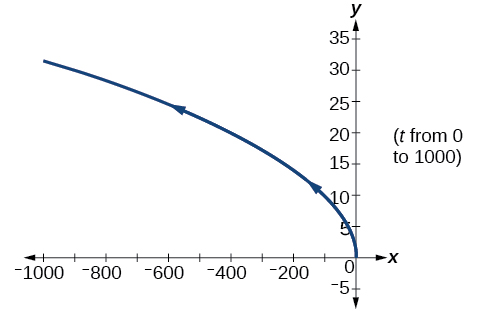$x=-2\mathrm{cos}\text{\hspace{0.17em}}t,\text{\hspace{0.17em}}y=6\text{\hspace{0.17em}}\mathrm{sin}\text{\hspace{0.17em}}t,\text{\hspace{0.17em}}0\le t\le \pi$

$x=-\mathrm{sec}\text{\hspace{0.17em}}t,\text{\hspace{0.17em}}y=\mathrm{tan}\text{\hspace{0.17em}}t,\text{\hspace{0.17em}}-\frac{\text{\hspace{0.17em}}\pi }{2}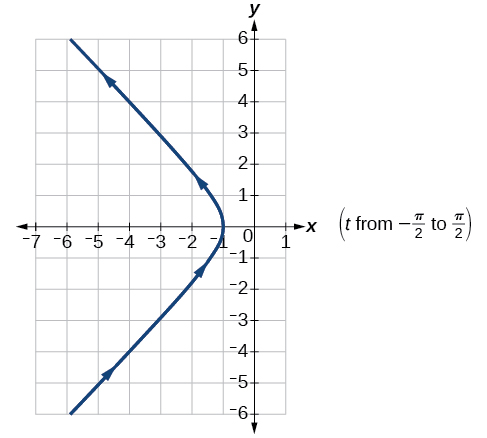For the following exercises, use the parametric equations for integers a and b :

$\begin{array}{l}x\left(t\right)=a\mathrm{cos}\left(\left(a+b\right)t\right)\\ y\left(t\right)=a\mathrm{cos}\left(\left(a-b\right)t\right)\end{array}$

Graph on the domain $\text{\hspace{0.17em}}\left[-\pi ,0\right],\text{\hspace{0.17em}}$ where $\text{\hspace{0.17em}}a=2\text{\hspace{0.17em}}$ and $\text{\hspace{0.17em}}b=1,\text{\hspace{0.17em}}$ and include the orientation.

Graph on the domain $\text{\hspace{0.17em}}\left[-\pi ,0\right],\text{\hspace{0.17em}}$ where $\text{\hspace{0.17em}}a=3\text{\hspace{0.17em}}$ and $\text{\hspace{0.17em}}b=2$ , and include the orientation.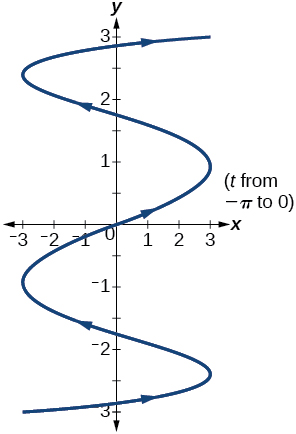Graph on the domain $\text{\hspace{0.17em}}\left[-\pi ,0\right],\text{\hspace{0.17em}}$ where $\text{\hspace{0.17em}}a=4\text{\hspace{0.17em}}$ and $\text{\hspace{0.17em}}b=3$ , and include the orientation.

A laser rangefinder is locked on a comet approaching Earth. The distance g(x), in kilometers, of the comet after x days, for x in the interval 0 to 30 days, is given by g(x)=250,000csc(π30x). Graph g(x) on the interval [0, 35]. Evaluate g(5)  and interpret the information. What is the minimum distance between the comet and Earth? When does this occur? To which constant in the equation does this correspond? Find and discuss the meaning of any vertical asymptotes.
The sequence is {1,-1,1-1.....} has
how can we solve this problem
Sin(A+B) = sinBcosA+cosBsinA
Prove it
Eseka
Eseka
hi
Joel
June needs 45 gallons of punch. 2 different coolers. Bigger cooler is 5 times as large as smaller cooler. How many gallons in each cooler?
7.5 and 37.5
Nando
find the sum of 28th term of the AP 3+10+17+---------
I think you should say "28 terms" instead of "28th term"
Vedant
the 28th term is 175
Nando
192
Kenneth
if sequence sn is a such that sn>0 for all n and lim sn=0than prove that lim (s1 s2............ sn) ke hole power n =n
write down the polynomial function with root 1/3,2,-3 with solution
if A and B are subspaces of V prove that (A+B)/B=A/(A-B)
write down the value of each of the following in surd form a)cos(-65°) b)sin(-180°)c)tan(225°)d)tan(135°)
Prove that (sinA/1-cosA - 1-cosA/sinA) (cosA/1-sinA - 1-sinA/cosA) = 4
what is the answer to dividing negative index
In a triangle ABC prove that. (b+c)cosA+(c+a)cosB+(a+b)cisC=a+b+c.
give me the waec 2019 questionsByByByBy Edward BitonBy